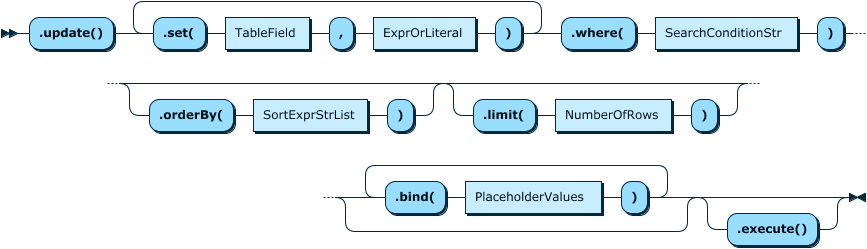Documentation Home
X DevAPI User Guide for MySQL Shell in Python Mode
PDF (US Ltr) - 1.2Mb
PDF (A4) - 1.2Mb

## 6.1 Syntax of the SQL CRUD Functions

The following SQL CRUD functions are available in X DevAPI.

### Table.insert()

The `Table.insert()` method works like an `INSERT` statement in SQL. It is used to store data in a relational table in the database. It is executed by the `execute()` function.

The following example shows how to use the `Table.insert() function`. The example assumes that the `test` schema exists and is assigned to the variable `db`, and that an empty table called `my_table` exists.

``````# Accessing an existing table
myTable = db.get_table('my_table')

# Insert a row of data.

Figure 6.1 Table.insert() Syntax Diagram### Table.select()

The `Table.select()` method works like a `SELECT` statement in SQL. Notice that `Table.select()` and `collection.find()` use different methods for sorting results: `Table.select()` uses the method `orderBy()`, reminiscent of the `ORDER BY` keyword in SQL, while the `sort()` method is used to sort the results returned by `Collection.find()`.

Figure 6.2 Table.select() Syntax Diagram### Table.update()

The `Table.update()` method works like an `UPDATE` statement in SQL.

Figure 6.3 Table.update() Syntax Diagram### Table.delete()

The `Table.delete()` method works like a `DELETE` statement in SQL.

Figure 6.4 Table.delete() Syntax Diagram